# Video Resource Dives into 3D World with Three.js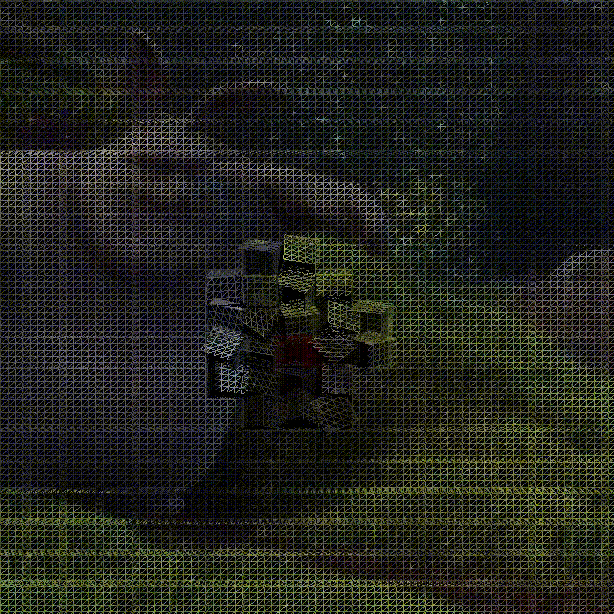## Background

The example I made a choice from Three.js repository is a video material. Using the material, We can import the video image into a 3D world. In this article, I will explain how I customize the example to my own idea. The key logic is a UV map for a geometry. Therefore, It may be informative at this example as well.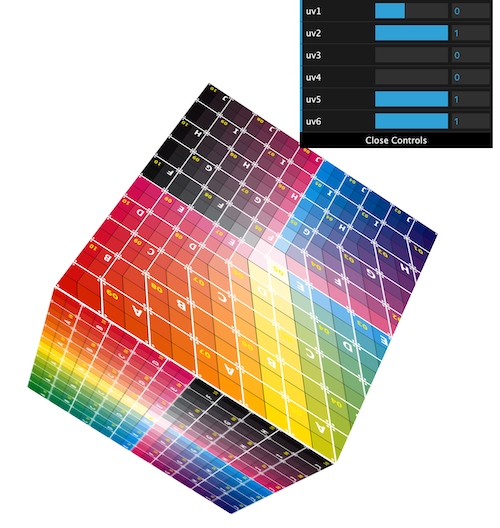#### Work Flow

• Create a scene, light, camera
• Create material
• Get video resource from video tag
• assign video resource with material
• Create geometries in grid shape
• change uv map
• Bind mouse over event to break video resolusions aroud its position
• Watch intersected object according to mouse

## Highlight Code

As I told in the top of the passage, the core code in my demonstration is to create a UV map in a geometry. But before going into the creation, take a look at how the video resource convert into video material. It is simply using THREE.VideoTexture.

video = document.getElementById('video');
texture = new THREE.VideoTexture(video);

In my test, I placed BoxGeometry in a grid in order to set the video resource on it. The code to put box objects in a grid is as follows.

var xgrid = 20, ygrid = 10;
var xsize = 480 / xgrid, ysize = 204 / ygrid;
var groupMesh = new THREE.Object3D();
for (i = 0; i < xgrid; i++) {
for (j = 0; j < ygrid; j++) {
geometry = new THREE.BoxBufferGeometry(xsize, ysize, xsize, 5, 5, 5);
material = new THREE.MeshLambertMaterial(parameters);
mesh = new THREE.Mesh(geometry, material);
// NOTE: Place mesh in a grid
mesh.position.x = (i - xgrid / 2) * xsize;
mesh.position.y = (j - ygrid / 2) * ysize;
mesh.position.z = 0;
mesh.scale.x = mesh.scale.y = mesh.scale.z = 1;
}
}

In this nested for loop, I create a box objects in each loop, then recreate a UV map of the geometry.

// NOT: normlized value for a grid.
var ux = 1 / xgrid;
var uy = 1 / ygrid;
for (var i = 0; i < xgrid; i++) {
for (var j = 0; j < ygrid; j++) {
change_uvs(geometry, ux, uy, i, j);
}
}
function change_uvs(geometry, unitx, unity, offsetx, offsety) {
var uvs = geometry.attributes.uv.array;
for (var i = 0; i < uvs.length; i += 2) {
uvs[i] = (uvs[i] + offsetx) * unitx;
uvs[i + 1] = (uvs[i + 1] + offsety) * unity;
}
}

What the uvs value in change_uvs function is how many faces in the geometry. Let’s take a BoxGeometry for example. If you define the default BoxGeometory, you can see it something like the following left image object. This basic geometry returns 48 value in return by geometry.attributes.uv.array. This value indicates 48 positions are including as a UV map. Why the calculation at 48 is:

var points = 4; // number of points in a face
var faces = 6; // number of faces, in this case 6 because of cube
var dimension = 2; number of dimension. UV is 2D map
var result = 4 * 6 * 2; // 48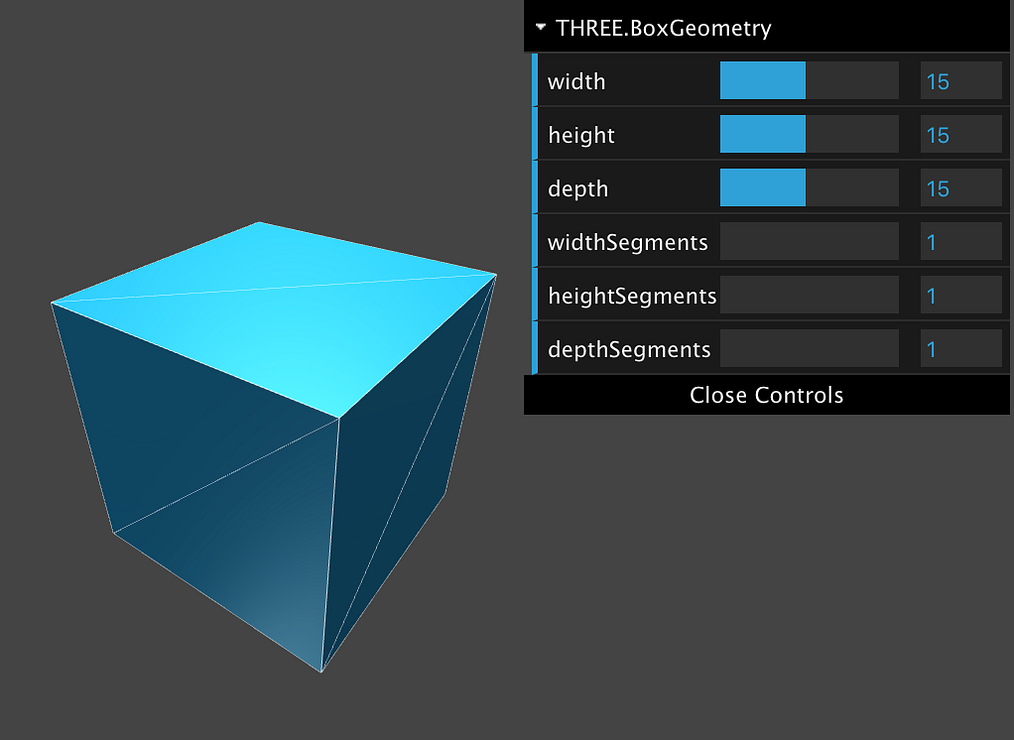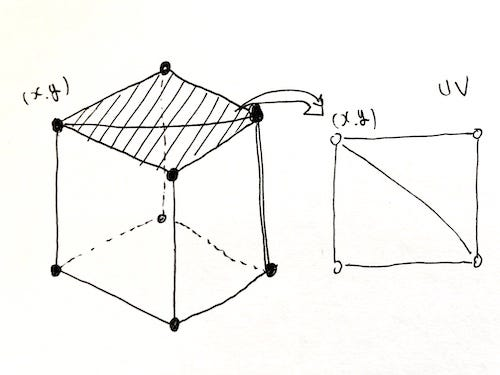If the width segments are 2, the result is 64.

var result = 6 * 64 * 2 + 4 * 2 * 2; // 64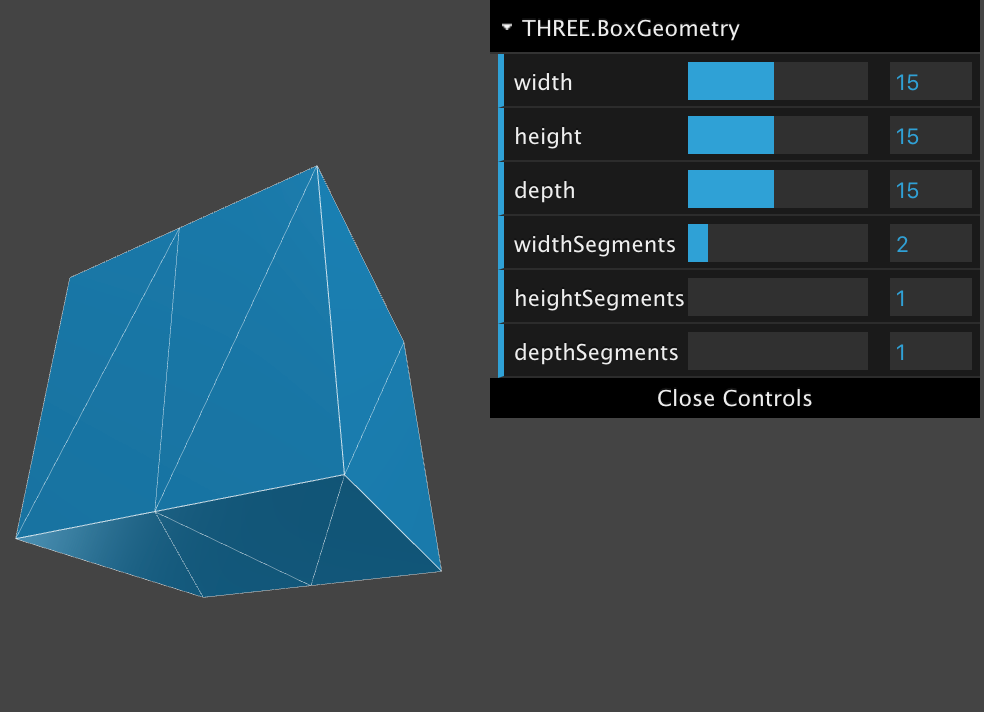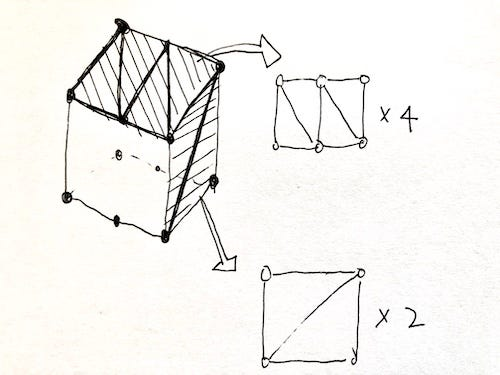The more segments are, the more fine-texture mesh and consume memories.

## Mouse Interaction

Now that I lined the cubes up, I define event listener for mouse interaction so as to rotate particular cubes according to a mouse position. The easy way to detect mouse position in 3D is using THREE.Raycaster. Naive use of the mouse position from the event is insufficient in 3D world. If it were scaling up, the mouse position would change at the end. You can get the point when the mouse intersects between a camera and a specific object. By setting an array that includes which objects you want to observe for intersection, you can get it.

var intersects = raycaster.intersectObjects(groupMesh.children);
if (intersects.length) {
const { x, y } = intersects.point;
const intersectObj = intersects.object;
}

In my customized example, I rotate objects whose coordinates are within a certain range around the mouse position.

for (var i = 0; i < cube_count; i++) {
mesh = groupMesh.children[i];
// NOTE: The range of circle to rotate object
const dist = mesh.position.distanceTo(intersects.point);
if (dist < 80) {
mesh.rotation.x += 10 * mesh.dx;
mesh.rotation.y += 10 * mesh.dy;
} else {
mesh.rotation.x = mesh.initialRotation.x;
mesh.rotation.y = mesh.initialRotation.y;
mesh.rotation.z = mesh.initialRotation.z;
}
}

https://codepen.io/mitsuya_bauhaus/pen/BaoxJGa

#### You may also like